# Reference entities: point, line, plane

## Introduction

A reference entity provides some support to the construction of the geometry without being taken into consideration during the mesh and solving operations. These entities are of « NO_EXIST » type.

It exists several “Reference” entities:

• Reference point
• Reference line defined between two points
• Reference plane

## When use it?

The table below presents when reference entities can be used.

Reference Entities When to use it ?
Reference point
• Symmetry of objects in relation to a reference point
• Creation of a reference line in relation to two reference points
Reference line
• Symmetry of objects in relation to a reference line
• Circular repetition of objects around a reference line
• Rotation of objects around a reference line
• Extrusion by revolution of faces around a reference line
• Helicoïdal extrusion of faces around a round a reference line
Reference plane
• Symmetry of objects in relation to a reference plane
• Cutting of objects in intersection with a reference plane
• Creation of a sketch on a reference plane

## How to create it?

The table below presents how the reference entities can be created.

Reference Entities How to create it?
Reference point

The creation of a reference point is identical to the creation of a standard point of Flux. It is necessary to define the coordinate system of creation and coordinates of the point ((X Y Z). To create a reference point:

• By menu: Construction > Reference > Reference point > New
• By icon: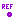Reference line

The creation of a reference line is identical to the creation of a standard line of Flux of segment type. It is necessary to define two points of the segment. These points can be standard points of objects or reference points previously created.

• By menu: Construction > Reference > Reference line > New
• By icon: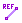Reference plane

The creation of a reference plane contains several type of definition (see below)

• By menu: Construction > Reference > Reference plane > New
• By icon: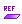## Type of reference plane

The table below shows different types of creation of a reference plane

Type Description Illustration
Defined by a face (flat) The reference plane is positioned on the plane of the face which must be flat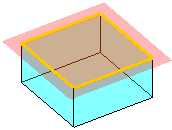Defined by a point and a line The reference plane is positioned on the plane describe by the selected point and both points of the selected line (the line must be a segment type)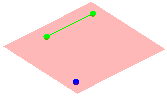Defined by 3 points The reference plane is positioned on the plane describe by 3 selected points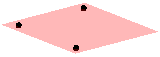Defined by a point and a line perpendicular The reference plane is positioned on the plane describe by the orthogonal projection on the trajectory of the selected line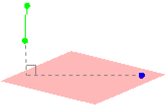XY plane with offset The reference plane is positioned parallel to the XY plane with an offset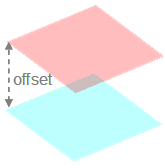XZ plane with offset The reference plane is positioned parallel to the XZ plane with an offset
YZ plane with offset The reference plane is positioned parallel to the YZ plane with an offset
Plane parallel to a plane The reference plane is positioned parallel to the selected reference plane with an offset
Defined by equation The reference plane is positioned on the plane describe by the equation Ax + Bx + Cx = D. The user must choose coefficients A, B, C and D
Defined by angle between two planes The reference plane is positioned with an angle in relation to the selected reference plane around an axis (the selected line)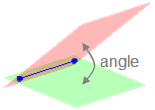## Predefined reference planes

Here is the list of predefined reference plane available at the opening of the modeler:

• XY plane
• XZ plane
• YZ plane

## Corresponding coordinate system

For each creation of reference plane (REFERENCEPLANE_1) a corresponding coordinate system (COORDSYS_REFERENCEPLANE_1) is created.